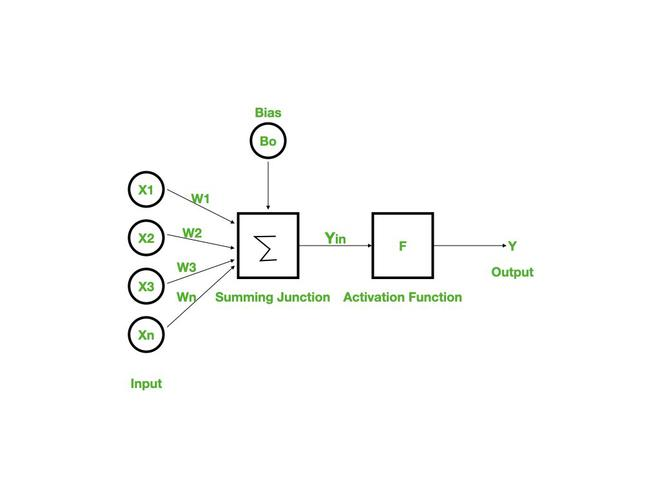# Backpropagation in Data Mining

• Last Updated : 24 Apr, 2022

Backpropagation is an algorithm that back propagates the errors from output nodes to the input nodes. Therefore, it is simply referred to as backward propagation of errors. It uses in the vast applications of neural networks in data mining like Character recognition, Signature verification, etc.

### Neural Network:

Neural networks are an information processing paradigm inspired by the human nervous system. Just like in the human nervous system, we have biological neurons in the same way in neural networks we have artificial neurons, artificial neurons are mathematical functions derived from biological neurons. The human brain is estimated to have about 10 billion neurons, each connected to an average of 10,000 other neurons. Each neuron receives a signal through a synapse, which controls the effect of the signal on the neuron.### Backpropagation:

Backpropagation is a widely used algorithm for training feedforward neural networks. It computes the gradient of the loss function with respect to the network weights and is very efficient, rather than naively directly computing the gradient with respect to each individual weight. This efficiency makes it possible to use gradient methods to train multi-layer networks and update weights to minimize loss; variants such as gradient descent or stochastic gradient descent are often used.

The backpropagation algorithm works by computing the gradient of the loss function with respect to each weight via the chain rule, computing the gradient layer by layer, and iterating backward from the last layer to avoid redundant computation of intermediate terms in the chain rule.

### Working of Backpropagation:

Neural networks use supervised learning to generate output vectors from input vectors that the network operates on. It Compares generated output to the desired output and generates an error report if the result does not match the generated output vector. Then it adjusts the weights according to the bug report to get your desired output.

### Backpropagation Algorithm:

Step 1: Inputs X, arrive through the preconnected path.

Step 2: The input is modeled using true weights W. Weights are usually chosen randomly.

Step 3: Calculate the output of each neuron from the input layer to the hidden layer to the output layer.

Step 4: Calculate the error in the outputs

`Backpropagation Error= Actual Output – Desired Output`

Step 5: From the output layer, go back to the hidden layer to adjust the weights to reduce the error.

Step 6: Repeat the process until the desired output is achieved.

### Need for Backpropagation:

Backpropagation is “backpropagation of errors” and is very useful for training neural networks. It’s fast, easy to implement, and simple. Backpropagation does not require any parameters to be set, except the number of inputs. Backpropagation is a flexible method because no prior knowledge of the network is required.

### Types of Backpropagation

There are two types of backpropagation networks.

• Static backpropagation: Static backpropagation is a network designed to map static inputs for static outputs. These types of networks are capable of solving static classification problems such as OCR (Optical Character Recognition).
• Recurrent backpropagation: Recursive backpropagation is another network used for fixed-point learning. Activation in recurrent backpropagation is feed-forward until a fixed value is reached. Static backpropagation provides an instant mapping, while recurrent backpropagation does not provide an instant mapping.

• It is simple, fast, and easy to program.
• Only numbers of the input are tuned, not any other parameter.
• It is Flexible and efficient.
• No need for users to learn any special functions.